# Radiation Source Localization¶

Author(s): Paul Miles, Jason Hite | Date Created: July 19, 2019

This example demonstrates how MCMC methods can be used to locate unknown sources of gamma radiation in an urban environment. For this demonstration we consider a simulated 250m x 178m block of downtown Washington D.C.

We utilize a highly simplified radiation transport model which ignores scattering. The model consists of the following components. There are $N_d$ gamma detectors located at positions $\mathbf{r}_i, i = 1, ..., N_d$. A ray tracing algorithm is implemented for a source located at $\mathbf{r}_0 = (x_0, y_0)$ with intensity $I_0$. Buildings affect the readings of the gamma detectors, so we have modeled their impact using a exponential decay function that depends on the building dimensions and average cross-section. For the $i^{th}$ detector, we let $N_i$ denote the number of buildings between $\mathbf{r}_0$ and $\mathbf{r}_i$. For $n_i = 1,...,N_i$, we let $s_{n_i}$ and $\sigma_{n_i}$ denote the length of the building segment and the average cross-section of the $n_i^{th}$ building, respectively. The surface area, efficiency, and measurement duration for the $i^{th}$ detector are denoted by $A_i, \varepsilon_i,$ and $\Delta t_i$, respectively. Finally, $B_i$ represents the expected background count rate at position $\mathbf{r}_i$. $$\Gamma_i = \frac{\Delta t_i\varepsilon_iA_iI_0}{4\pi|\mathbf{r}_i - \mathbf{r}_0|^2}\exp\Big(-\sum_{n_i=1}^{N_i}\sigma_{n_i}s_{n_i}\Big) + E[B_i\Delta t_i]$$

This model is implemented in the open source Python package gefry3. The input deck for this example can be found here. For additional reading on this area of research, please refer to the following articles:

• Hite, J., & Mattingly, J. (2018). Bayesian Metropolis Methods for Source Localization in an Urban Environment. Radiation Physics and Chemistry. https://doi.org/10.1016/j.radphyschem.2018.06.024
• Ştefănescu, R., Schmidt, K., Hite, J., Smith, R. C., & Mattingly, J. (2017). Hybrid optimization and Bayesian inference techniques for a non‐smooth radiation detection problem. International Journal for Numerical Methods in Engineering, 111(10), 955-982. https://doi.org/10.1002/nme.5491
• Hite, J. M., Mattingly, J. K., Schmidt, K. L., Ştefănescu, R., & Smith, R. (2016, September). Bayesian metropolis methods applied to sensor networks for radiation source localization. In Multisensor Fusion and Integration for Intelligent Systems (MFI), 2016 IEEE International Conference on (pp. 389-393). IEEE. https://doi.org/10.1109/MFI.2016.7849519

Acknowledgment: Funding for this research provided by the Consortium for Nonproliferation Enabling Capabilities (CNEC).

Package Installation: All packages can be install via

pip install package_name


with the exception of gefry3, which requires

pip install git+https://github.com/jasonmhite/gefry3


Advanced statistical plots are generated using the mcmcplot package.

To run this particular notebook, please execute the two cells below. The first cell will install gefry3 and the second descartes.

In [ ]:
pip install git+https://github.com/jasonmhite/gefry3

In [ ]:
pip install descartes

In :
# import required packages
import os
import gefry3
import numpy as np
from pymcmcstat.MCMC import MCMC
from descartes.patch import PolygonPatch
import seaborn as sns
import matplotlib.pyplot as plt
np.seterr(over = 'ignore')
NOBS = 1 # number of observations for each detector
NS = int(1e3) # number of MCMC simulations


# Define Radiation Model, Problem Domain, and Background Radiation¶

In :
# Setup Radiation Model - Downtown Urban Environment Simulation
P = gefry3.read_input_problem('data_files' + os.sep + 'g3_deck.yml', 'Simple_Problem')
# Setup background
nominal_background = 300
dwell = np.array([detector.dwell for detector in P.detectors])
B0 = nominal_background * dwell
# True source and intensity
S0 = P.source.R  # m
I0 = P.source.I0  # Bq
nominal = P(S0, I0)
ndet = len(P.detectors)
# Define observations
observations = np.random.poisson(
nominal + nominal_background * dwell,
size=(
NOBS,
ndet,
),
)
observations = observations.astype(np.float64)
# Setup parameter bounds
XMIN, YMIN, XMAX, YMAX = P.domain.all.bounds
IMIN, IMAX = I0 * 0.1, I0 * 10
XMIN, YMIN, XMAX, YMAX = P.domain.all.bounds
print(observations)
print('S0 = {} m, I0 = {} Bq'.format(S0, I0))

[[1522. 1544. 1475. 1682. 1602. 1517. 1546. 1769. 1664. 1668.]]
S0 = [158.  98.] m, I0 = 3214000000.0 Bq


# Define Sum-of-Squares Error Function.¶

In :
# Radiation Sum of Squares Function
x, y, I = theta
model, background = data.user_defined_object
output = model((x, y), I) + background

res = data.ydata - output
ss = (res ** 2).sum(axis = 0)
return ss


# Initialize MCMC Object and Setup Simulation¶

Note, we pass the radiation model into our residual function via the data structure. Also note, the background is included as a parameter, but it is not sampled during the simulation.

In :
# Initialize MCMC object
mcstat = MCMC()
# setup data structure for dram
x=np.zeros(observations.shape),
y=observations,
user_defined_object=[
P,
B0,
],
)
name='$x_0$',
theta0=140.,
minimum=XMIN,
maximum=XMAX,
)
name='$y_0$',
theta0=85.,
minimum=YMIN,
maximum=YMAX,
)
name='$I_0$',
theta0=3.0e9,
minimum=IMIN,
maximum=IMAX
)

mcstat.simulation_options.define_simulation_options(
nsimu=NS,
method='dram',
verbosity=1,
waitbar=1,
save_to_json=False,
save_to_bin=False,
)

mcstat.model_settings.define_model_settings(
sigma2=observations.sum(axis=0),
)


# Run Simulation¶

In :
# Run mcmcrun
mcstat.run_simulation()

Sampling these parameters:
name      start [      min,       max] N(       mu,   sigma^2)
$x_0$:    140.00 [ 0.00e+00,    246.62] N( 0.00e+00,      inf)
$y_0$:     85.00 [ 0.00e+00,    176.33] N( 0.00e+00,      inf)
$I_0$:  3.00e+09 [ 3.21e+08,  3.21e+10] N( 0.00e+00,      inf)
[-----------------100%-----------------] 1000 of 1000 complete in 66.6 sec

# Process Results and Display Statistics¶

In :
from pymcmcstat import mcmcplot as mcp
import seaborn as sns

results = mcstat.simulation_results.results
# specify burnin period
burnin = int(results['nsimu']/2)
# display chain statistics
chain = results['chain'][burnin:, :]
sschain = results['sschain'][burnin:, :]
names = results['names']
mcstat.chainstats(chain, results)
print('Acceptance rate: {:6.4}%'.format(100*(1 - results['total_rejected'])))
print('Model Evaluations: {}'.format(results['nsimu']
- results['iacce']
+ results['nsimu']))
# generate mcmc plots
sns.set_style('white')
mcp.plot_density_panel(chain, names)
mcp.plot_chain_panel(chain, names)
mcp.plot_pairwise_correlation_panel(chain, names)

------------------------------
name      :       mean        std     MC_err        tau     geweke
$x_0$     :   160.6402     2.7195     0.3393    10.0607     0.9957
$y_0$     :    98.9279     1.8359     0.1848     6.0494     0.9936
$I_0$     :  2.406e+09  3.638e+08 48017969.7110    11.4787     0.9392
------------------------------
==============================
Acceptance rate information
---------------
Results dictionary:
Stage 1: 20.30%
Stage 2: 56.10%
Net    : 76.40% -> 764/1000
---------------
Chain provided:
Net    : 80.00% -> 400/500
---------------
Note, the net acceptance rate from the results dictionary
may be different if you only provided a subset of the chain,
e.g., removed the first part for burnin-in.
------------------------------
Acceptance rate:   76.4%
Model Evaluations: 1797

Out: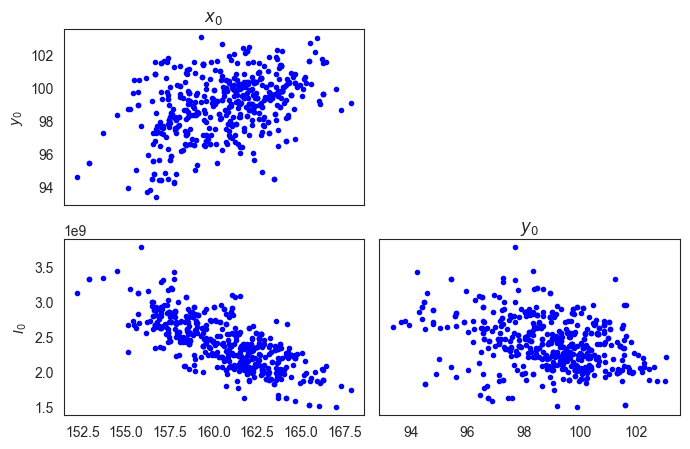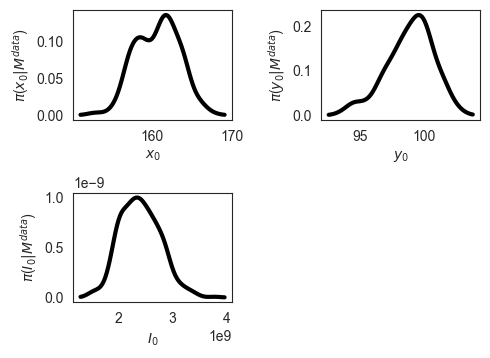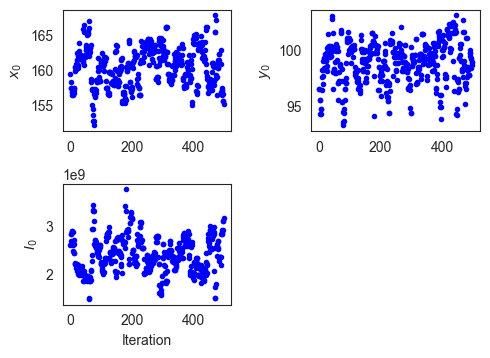# Generate Plot of Urban Environment With Source Location Distributions¶

First we define a function to allow us to add axes within a figure.

In :
def add_subplot_axes(ax,rect,**kwargs):
fig = plt.gcf()
box = ax.get_position()
width = box.width
height = box.height
inax_position  = ax.transAxes.transform(rect[0:2])
transFigure = fig.transFigure.inverted()
infig_position = transFigure.transform(inax_position)
x = infig_position
y = infig_position
width *= rect
height *= rect  # <= Typo was here
x_labelsize = subax.get_xticklabels().get_size()
y_labelsize = subax.get_yticklabels().get_size()
x_labelsize *= rect**0.5
y_labelsize *= rect**0.5
subax.xaxis.set_tick_params(labelsize=x_labelsize)
subax.yaxis.set_tick_params(labelsize=y_labelsize)
return subax


We set the context of our displays to "talk" which will improve their visibility for presentation. To begin, we will plot the joint distribution relative to the true source location.

In :
sns.set_context('talk')
settings = dict(jointplot=dict(kind='kde', color='b'),
marginal_kws=dict(alpha=1))
fig0 = mcp.plot_joint_distributions(
chain[:, 0:2],
names,
settings=settings
);
fig0.ax_joint.plot(P.source.R, P.source.R, 'or',
linewidth=3, markerfacecolor='r',
markersize=10, label='True Source');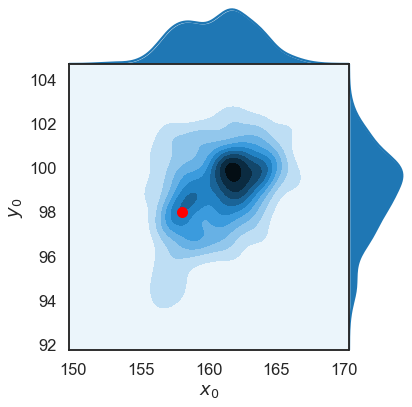Next, we plot the joint distributions relative to our entire simulated urban environment. We have added an insert to highlight the relative positioning of the posterior distributions and the true source location.

In :
settings = dict(jointplot=dict(kind='kde', color='b'),
marginal_kws=dict(alpha=1))
fig = mcp.plot_joint_distributions(
chain[:, 0:2],
names,
settings=settings);
fig.ax_marg_x.set_xlim([XMIN, XMAX])
fig.ax_marg_y.set_ylim([YMIN, YMAX])
ax = fig.ax_joint
ax.plot(P.source.R, P.source.R,
linewidth=3,
markerfacecolor='r',
markersize=8,
marker='*')
# add buildings to plot
for building in P.domain.solids:
patch = PolygonPatch(building.geom, alpha=0.3,
facecolor='g', edgecolor='k')
# Create insert
rect = [0.1, 0.1, 0.3, 0.3]
ax1 = add_subplot_axes(ax, rect, facecolor='w')
sns.kdeplot(chain[:, 0], chain[:, 1], ax=ax1, color='b')
mu = results['mean']
xlimits = [mu - 7.5, mu + 7.5]
ylimits = [mu - 7.5, mu + 7.5]
ax1.set_xlim(xlimits)
ax1.set_ylim(ylimits)
ax1.plot(P.source.R, P.source.R,
linewidth=3,
markerfacecolor='r',
markersize=20,
marker='*')
# add buildings to plot
for building in P.domain.solids:
patch = PolygonPatch(building.geom, alpha=0.3,
facecolor='g', edgecolor='k')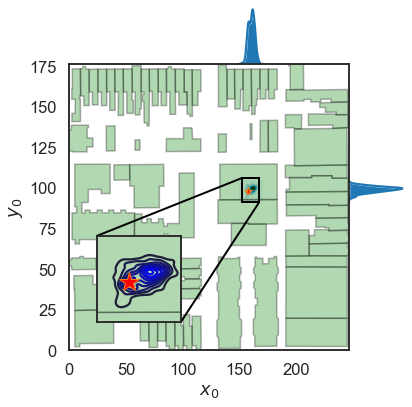We observe that the poster distributions for the source location are within a couple meters of the true source location (red star - $R_{true} = [158, 98]$ meters). Furthermore, the true location is within the uncertainty bounds of our posteriors.